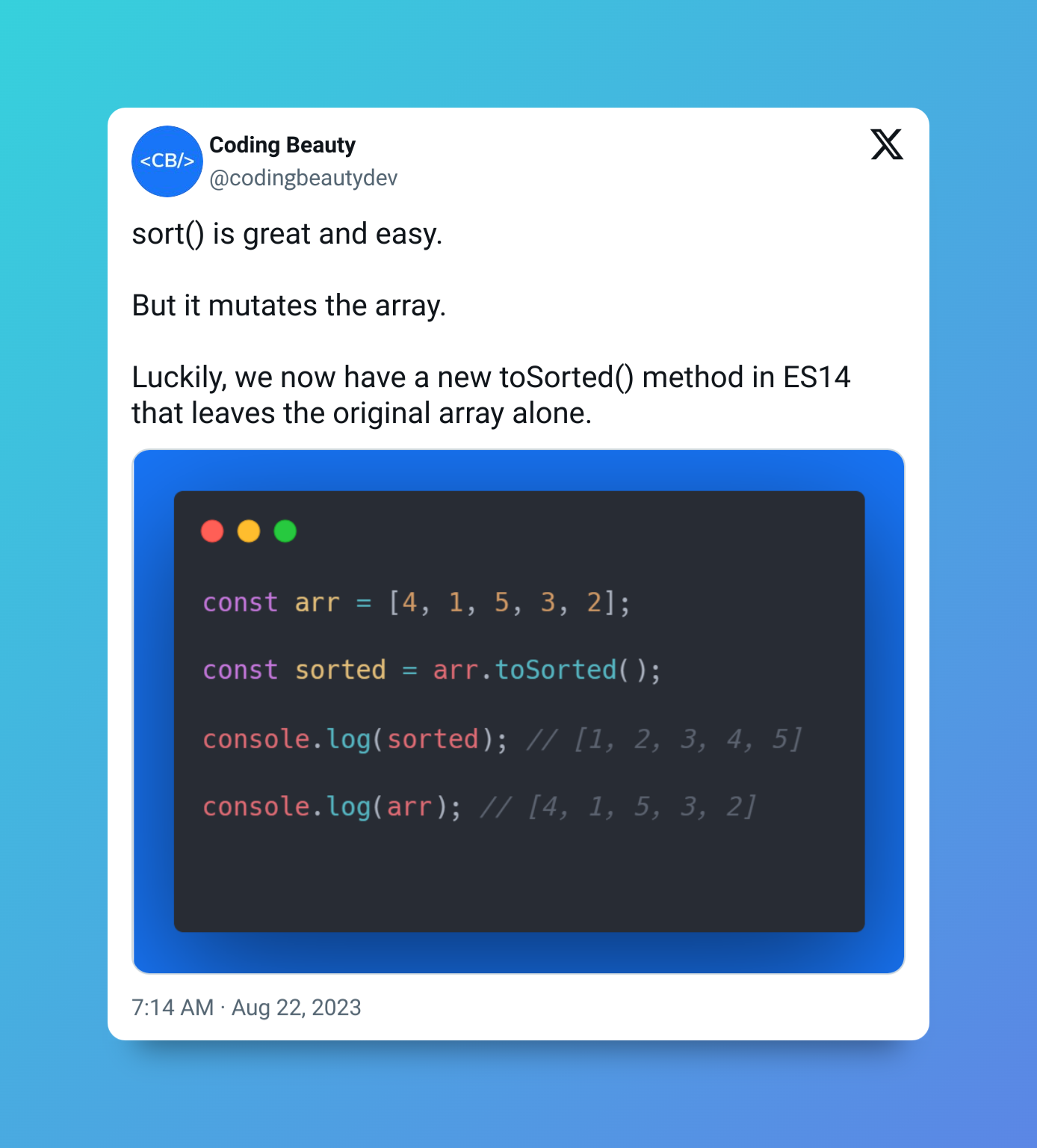# How to Get the Sum of an Array in JavaScript

To get the sum of an array in JavaScript, call the `reduce()` method on the array, with two arguments: a callback that takes two arguments and returns their sum, and `0`.

For example:

JavaScript
``````const sum = (arr) => arr.reduce((a, b) => a + b, 0);

const arr = [1, 2, 3, 4, 5]
const result = sum(arr);
console.log(arr); // 15``````

When we call `reduce()` method on `arr`, it loops through the array and adds each element to a sum value When the loop is done, this value will hold the total sum of the array, and `reduce()` will return it.

The callback argument is called on each array element and takes two arguments, a and b. a represents the total sum value so far and `b` represents the current element in the iteration. The callback simply adds the current element to the total sum value so far and returns it.

The second argument `reduce()` takes is an initial value of the total sum. We set it to `0` in this case, but this is the default value anyway, so we can leave it out:

JavaScript
``````// No need for second argument when it's zero
const sum = (arr) => arr.reduce((a, b) => a + b);

const arr = [1, 2, 3, 4, 5]
const result = sum(arr);
console.log(arr); // 15``````## Get sum of array with `for..of` loop

Alternatively, we can get the sum of an array in JavaScript using a `for..of` loop and taking these steps:

1. Create a variable to store the sum.
2. Loop over the array.
3. Add each element to the sum.

For example:

JavaScript
``````function sum(arr) {
let result = 0;
for (const item of arr) {
result += item;
}
return result;
}

const arr = [1, 2, 3, 4, 5]
const result = sum(arr);
console.log(arr); // 15``````

Throughout the loop, `item` will hold the value of each array element, so we add its value to `sum` in each iteration.

## Get sum of numbers with `forEach()` method

Of course, wherever we can use `for..of` we can use `forEach()`:

JavaScript
``````function sum(arr) {
let result = 0;
arr.forEach(item => {
result += item;
});
return result;
}

const arr = [1, 2, 3, 4, 5]
const result = sum(arr);
console.log(result); // 15
``````

The `forEach()` loops over each element in the `arr` array. It takes a callback function as its argument that is called on each element of the array. Like we did with `for..of`, the callback function simply adds the current element to the `result` variable.

## Get sum of numbers with `for` loop

And `forEach()` or `for...of` can equally be replaced with the traditional `for` loop:

JavaScript
``````function sum(arr) {
let result = 0;
for (let i = 0; i < arr.length; i++) {
result += arr[i];
}
return result;
}

const arr = [1, 2, 3, 4, 5]
const result = sum(arr);
console.log(result); // 15
``````

## How do they compare in speed?

We performed a test to compare the running times of the four different methods above for summing an array in JavaScript: the `for` loop, `forEach()`, `for...of` loop, and `reduce()` methods.

We tested each method three times and noted how long it took to complete each test. Then we averaged the results to get a more accurate representation of the execution times.

Each test case involved summing an array of the first `1` million positive integers.

The `for` loop method was the fastest, taking only an average of 4.530 ms to complete. The `forEach()` method was the slowest, with an average execution time of 31.123 ms. The `for...of` loop and `reduce()` methods were relatively close in execution time, with average execution times of 22.940 ms and 21.463 ms, respectively.

Overall, the `for` loop method was the most efficient for summing an array in JavaScript, while the `forEach()` method was the least efficient. Still, the best method to use depends on your specific use case and the array’s size.

When dealing with small arrays, the difference in performance between different methods for summing the array may not be noticeable. But as the size of the array increases, this difference can become more significant and can affect the code’s overall running time.

So for very large arrays, it’s important that we choose the most efficient method for computing the sum to avoid slow execution times. By choosing the most efficient method, we’ll make sure that our code runs as quickly and efficiently as possible, even when dealing with huge amounts of data.

## 11 Amazing New JavaScript Features in ES13

This guide will bring you up to speed with all the latest features added in ECMAScript 13. These powerful new features will modernize your JavaScript with shorter and more expressive code.